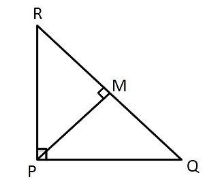Courses
Courses for Kids
Free study material
Free LIVE classes
MoreLIVE
Join Vedantu’s FREE Mastercalss

# PQR is a triangle right angled at P and M is a point on QR such that PM $\bot$ QR, Show that $P{M^2} = QM \cdot MR$Verified
264.3k+ views
Hint: For the right angled triangle, we have to use Pythagoras theorem to get the desired solution.

From the given information, if we draw a triangle it is similar to the figure below.In $\vartriangle PMR,$ by Pythagoras theorem,
${\left( {PR} \right)^2} = {\left( {PM} \right)^2} + {\left( {RM} \right)^2}$ .... (1)
In $\vartriangle PMQ,$by Pythagoras theorem,
${\left( {PQ} \right)^2} = {\left( {PM} \right)^2} + {\left( {MQ} \right)^2}$ .... (2)
In $\vartriangle PQR,$ by Pythagoras theorem,
${\left( {RQ} \right)^2} = {\left( {RP} \right)^2} + {\left( {PQ} \right)^2}$ .... (3)
$\therefore {\left( {RM + MQ} \right)^2} = {\left( {RP} \right)^2} + {\left( {PQ} \right)^2}$
$\therefore {\left( {RM + MQ} \right)^2} + 2RM \cdot MQ = {(RP)^2} + {(PQ)^2}$ .... (4)
Adding equation (1) and (2) we get,
${\left( {PR} \right)^2} + {\left( {PQ} \right)^2} = 2{\left( {PM} \right)^2} + {\left( {RM} \right)^2} + {\left( {MQ} \right)^2}$ .... (5)
From equations (4) and (5), we get
$2RM \cdot MQ = 2{\left( {PM} \right)^2}$
$\therefore {\left( {PM} \right)^2} = RM.MQ$
Hence proved.
Note:If a perpendicular is drawn from the vertex of the right angle to the hypotenuse then triangles on both sides of the perpendicular are similar to the whole triangle and to each other. If two triangles are similar, then the ratio of their corresponding sides are equal.
Last updated date: 26th Sep 2023
Total views: 264.3k
Views today: 2.64k